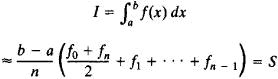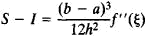# Trapezoidal Rule

Also found in: Wikipedia.

## trapezoidal rule

[¦trap·ə¦zȯid·əl ′rül]
(mathematics)
The rule that the integral from a to b of a real function ƒ(x) is approximated by where x0= a, xj = xj-1+ (b - a)/ n for j = 1, 2, …, n - 1.
McGraw-Hill Dictionary of Scientific & Technical Terms, 6E, Copyright © 2003 by The McGraw-Hill Companies, Inc.
The following article is from The Great Soviet Encyclopedia (1979). It might be outdated or ideologically biased.

## Trapezoidal Rule

(or trapezoid rule), a formula for the approximate evaluation of definite integrals. It has the formwhere fm = f(a + mh), h = (ba)/n, and m = 0, 1, . . . ., n.

The use of the trapezoidal rule may be understood in geometric terms by regarding the definite integral I as expressing the area under the curve y = f(x) from x = a to x = b—that is, the area of the region bounded by the segment on the x-axis between the points a and b, the perpendiculars to the x-axis at these points (the lengths of the perpendiculars are given by the ordinates f0 and fn), and the graph of f(x). In applying the trapezoidal rule, we replace this area by the sum of the areas of the trapezoids the lengths of whose bases are given by the pairs of ordinates fm, fm + 1 (m = 0, 1, . . ., n – 1).

The error resulting from the use of the trapezoidal rule iswhere a ≤ ξ ≤ b. Formulas of greater accuracy for the approxi mate evaluation of definite integrals are discussed in APPROXIMATE INTEGRATION.

References in periodicals archive ?
Error bounds for the trapezoidal rule. The integrand of the integral (4.2) is periodic if the parametrization function [phi] is periodic, e.g., a circle as in (4.3).
Day  proposed Newton-Cotes integration formula of the trapezoidal rule for the solutions of outer and inner integral to obtain approximate solutions of integrodifferential equations.
Trapezoidal rule was used for calculation of AUC0-48 and AUC0-[?] and it was found to be 208.77 h/g/l and 208.74 h/g/l respectively.
For the average temperature, the plain trapezoidal rule ([H.sub.0,0] approximation) is employed in all layers, except for the innermost layer, where the second-order two-side corrected trapezoidal rule ([H.sub.2,1] approximation) is utilized.
To test different numerical integration methods, needed for Dirlik's method, the Midpoint Rule, Trapezoidal Rule and Simpson's Rule were compared by integrating over a Rayleigh distribution for various probabilities from .001 to .999999999.
Each iteration of the iterative method requires solving an n x n linear system which is obtained by discretizing the integrals in the integral equation by the trapezoidal rule. The linear system is solved by a combination of the generalized minimal residual (GMRES) method and the fast multipole method (FMM) in 0(n ln n) operations.
Thermally induced residual stresses and bending moments are calculated by using the trapezoidal rule.
In particular, students would be familiar with the midpoint rule, the elementary trapezoidal rule and Simpson's rule.
The straight forward computation of (7) by the trapezoidal rule leads to an O([[OMEGA].sup.4]) algorithm.
Under the assumption of Theorem 3.1, the following perturbed trapezoidal rule also holds:

Site: Follow: Share:
Open / Close